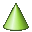# KK Card

The KK card defines a mesh of surface triangles in the shape of a conical section.

On the Construct tab, in the Surfaces group, click theCone (KK) icon.

## Parameters:

S1
The start point of the axis of the cone (the centre of the base).
S2
The end point of the axis (the tip of the cone, or the centre point of the circle when creating a conical section).
S3
A point on the radius of the base.
S4
If this parameter is defined, the cone is cut off at the top. If not defined the cone will have a sharp tip. This point must be in the plane given by S1, S2 and S3.
Start angle at the bottom
The angle $\phi$ from the plane S2-S1-S3 at which the bottom of the cone should start.
End angle at the bottom
The angle $\phi$ from the plane S2-S1-S3 at which the bottom of the cone should end.
Start angle at the top
The angle $\phi$ from the plane S2-S1-S3 at which the top of the cone should start.
End angle at the top
The angle $\phi$ from the plane S2-S1-S3 at which the top of the cone should end.
Maximum edge length (bottom)
The maximum edge length of the triangles along the base arc (in the plane containing S1) of the cone. This value is in m and is scaled by the SF card. If this parameter is left empty, the value specified with the IP card is used.
Maximum edge length (top)
This value only applies if S4 is specified and gives the maximum edge length of the triangles along the top arc (in the plane containing S2) of the cone. This value is in m and is scaled by the SF card. If this parameter is left empty, the value specified with the IP card is used.
Normal vector directed
The triangles can be created so that the normal vector points Outward (away from the axis) or Inward (to the inside of the cone).
Scale second half axis
If this parameter is empty or is set to 1, a cone with a circular cross section is created. If set to $\frac{\text{b}}{a}$ , a cone with an elliptical cross section is created. Here $\frac{\text{b}}{a}$ gives the ratio of the two half axes, where a is the distance S1–S3. It is recommended to generate elliptical cones with extremely small or extremely large axial ratios with a CAD system as the distortion formulation used in PREFEKO may fail in these cases.

The fineness of the mesh on the shell’s surface is determined by the maximum edge length specified by the last IP card prior to the KK card. Along the arcs, accurate modelling of the geometry may require finer segmentation and the values Maximum edge length (bottom) and Maximum edge length (top) specify the maximum edge length along the corresponding arcs. Maximum edge length (top) is only used when a truncated cone is created. If either of these values is not specified the length specified with the IP card will be used on the corresponding arc.

## Examples of KK card usage

All of the following meshes are created using the KK card. These examples show a sharp cone, an oblique elliptical cone, a conical section with different angles at the top and bottom as well as a conical section where the start angle is not in the plane S2–S1–S3.